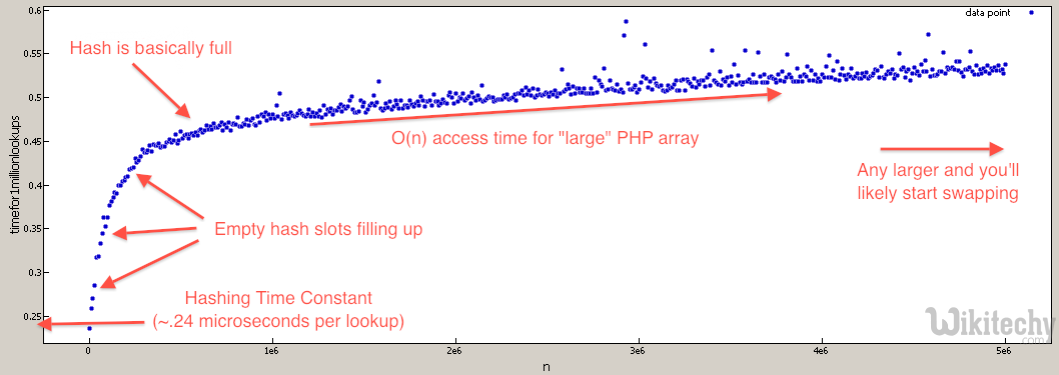PHP

# [ Solved – 1 Answers ] PHP – List of Big-O for PHP functions

List of Big-O for PHP functions After using PHP for a while now, we noticed that not all PHP built in functions as fast
• After using PHP for a while, we noticed that not all PHP built in functions as fast as expected.
• Consider the below two possible implementations of a function that finds if a number is prime using a cached array of primes.
Php Code
``````//very slow for large \$prime_array
\$prime_array = array( 2, 3, 5, 7, 11, 13, .... 104729, ... );
\$result_array = array();
foreach( \$prime_array => \$number ) {
\$result_array[\$number] = in_array( \$number, \$large_prime_array );
}

//speed is much less dependent on size of \$prime_array, and runs much faster.
\$prime_array => array( 2 => NULL, 3 => NULL, 5 => NULL, 7 => NULL,
11 => NULL, 13 => NULL, .... 104729 => NULL, ... );
foreach( \$prime_array => \$number ) {
\$result_array[\$number] = array_key_exists( \$number, \$large_prime_array );
}``````
• This is because in_array is implemented with a linear search O(n) which will linearly slow down as \$prime_array grows. Where the array_key_exists function is implemented with a hash lookup O(1) which will not slow down unless the hash table gets extremely populated (in which case it’s only O(n)).
• So far we had to discover the big-O’s via trial and error, and occasionally looking at the source code. Now for the question…

Is there was a list of the theoretical (or practical) big O times for all* the PHP built in functions?

*or at least the interesting ones

For example find it very hard to predict what the big O of functions listed because the possible implementation depends on unknown core data structures of PHP: array_merge, array_merge_recursive, array_reverse, array_intersect, array_combine, str_replace (with array inputs), etc.

• We gone though and either via benchmark or code-skimming to characterize the array_* functions.

Note:

• All the Big-O where calculated assuming a hash lookup is O(1) even though it’s really O(n).
• The coefficient of the n is so low, the ram overhead of storing a large enough array would hurt you before the characteristics of lookup Big-O would start taking effect.
• For example the difference between a call to array_key_exists at N=1 and N=1,000,000 is ~50% time increase.

#### Interesting Points:

1. isset/array_key_exists is much faster than in_array and array_search
2. +(union) is a bit faster than array_merge (and looks nicer). But it does work differently so keep that in mind.
3. shuffle is on the same Big-O tier as array_rand
4. array_pop/array_push is faster than array_shift/array_unshift due to re-index penalty

#### Lookups:

• array_key_exists O(n) but really close to O(1) – this is because of linear polling in collisions, but because the chance of collisions is very small, the coefficient is also very small. I find you treat hash lookups as O(1) to give a more realistic big-O. For example the different between N=1000 and N=100000 is only about 50% slow down.
• isset( \$array[\$index] ) O(n) but really close to O(1) – it uses the same lookup as array_key_exists. Since it’s language construct, will cache the lookup if the key is hardcoded, resulting in speed up in cases where the same key is used repeatedly.
• in_array O(n) – this is because it does a linear search though the array until it finds the value.
• array_search O(n) – it uses the same core function as in_array but returns value.

#### Queue functions:

• array_push O(∑ var_i, for all i)
• array_pop O(1)
• array_shift O(n) – it has to reindex all the keys
• array_unshift O(n + ∑ var_i, for all i) – it has to reindex all the keys

#### Array Intersection, Union, Subtraction:

• array_intersect_key if intersection 100% do O(Max(param_i_size)*∑param_i_count, for all i), if intersection 0% intersect O(∑param_i_size, for all i)
• array_intersect if intersection 100% do O(n^2*∑param_i_count, for all i), if intersection 0% intersect O(n^2)
• array_intersect_assoc if intersection 100% do O(Max(param_i_size)*∑param_i_count, for all i), if intersection 0% intersect O(∑param_i_size, for all i)
• array_diff O(π param_i_size, for all i) – That’s product of all the param_sizes
• array_diff_key O(∑ param_i_size, for i != 1) – this is because we don’t need to iterate over the first array.
• array_merge O( ∑ array_i, i != 1 ) – doesn’t need to iterate over the first array
• + (union) O(n), where n is size of the 2nd array (ie array_first + array_second) – less overhead than array_merge since it doesn’t have to renumber
• array_replace O( ∑ array_i, for all i )

#### Random:

• shuffle O(n)
• array_rand O(n) – Requires a linear poll.

Obvious Big-O:

• array_fill O(n)
• array_fill_keys O(n)
• range O(n)
• array_splice O(offset + length)
• array_slice O(offset + length) or O(n) if length = NULL
• array_keys O(n)
• array_values O(n)
• array_reverse O(n)

EDIT:

• For those who doubt that PHP array lookups are O(N), we written a benchmark to test that (they are still effectively O(1) for most realistic values).Php Code
``````\$tests = 1000000;
\$max = 5000001;
for( \$i = 1; \$i <= \$max; \$i += 10000 ) {
//create lookup array
\$array = array_fill( 0, \$i, NULL );

//build test indexes
\$test_indexes = array();
for( \$j = 0; \$j < \$tests; \$j++ ) {
\$test_indexes[] = rand( 0, \$i-1 );
}

//benchmark array lookups
\$start = microtime( TRUE );
foreach( \$test_indexes as \$test_index ) {
\$value = \$array[ \$test_index ];
unset( \$value );
}
\$stop = microtime( TRUE );
unset( \$array, \$test_indexes, \$test_index );
printf( "%d,%1.15f\n", \$i, \$stop - \$start ); //time per 1mil lookups
unset( \$stop, \$start );
}``````